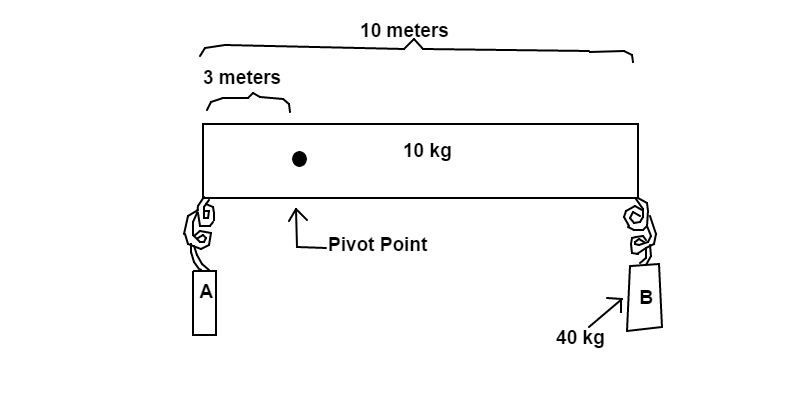# Stationary Stone BarA uniform stone bar with a mass of $10\text{ kg}$ and a length of $10\text{ m}$ has a pivot point that is $3\text{ m}$ away from the nearest edge. Two weights, $A$ and $B$, are hung onto the edges of the bar by massless springs where weight $B$ is hung on the edge farthest from the pivot point. If the mass of weight $B$ is $40\text{ kg}$, what must the mass of weight $A$ be to prevent the bar from turning?

Assume $g=9.81 \dfrac{\text m}{\text s^2}$.

×